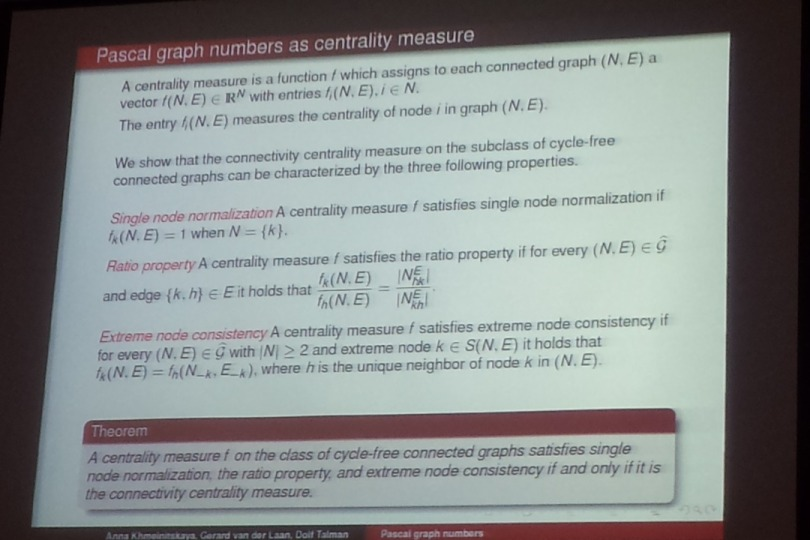• A
• A
• A
• ABC
• ABC
• ABC
• А
• А
• А
• А
• А
Regular version of the site

All-Russian seminar "Mathematical methods of decision analysis in economics, finance and politics" was held

Speaker: Anna Khmelnitskaya (University of Twente, The Netherlands)
Title: Generalization of binomial coefficients to numbers on the nodes of graphsCoauthored with Gerard van der Laan from VU University Amsterdam, and Dolf Talman from Tilburg University

Abstract
:

The triangular array of binomial coefficients, or Pascal's triangle, is formed by starting with an apex of 1. Every row of Pascal's triangle can be seen as a line-graph, to each node of which the corresponding binomial coefficient is assigned. We show that the binomial coefficient of a node is equal to the number of ways the line-graph can be constructed when starting with this node and adding subsequently neighboring nodes one by one. Using this interpretation we generalize the sequences of binomial coefficients on each row of Pascal's triangle to so-called Pascal graph numbers assigned to the nodes of an arbitrary (connected) graph. We show that on the class of connected cycle-free graphs the Pascal graph numbers have properties that are very similar to the properties of binomial coefficients. We also show that for a given connected cycle-free graph the Pascal graph numbers, when normalized to sum up to one, are equal to the steady state probabilities of some Markov process on the nodes. Properties of the Pascal graph numbers for connected graphs which are not cycle-free are also discussed. Because of the Pascal graph number of a node in a connected graph is defined as the number of ways the graph can be constructed by a sequence of increasing connected subgraphs starting from this node, the Pascal graph numbers can be seen as a measure of centrality in the graph.